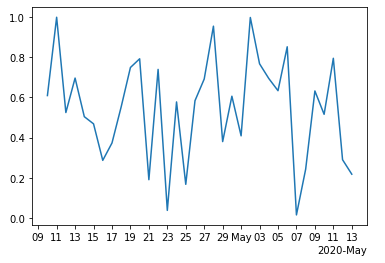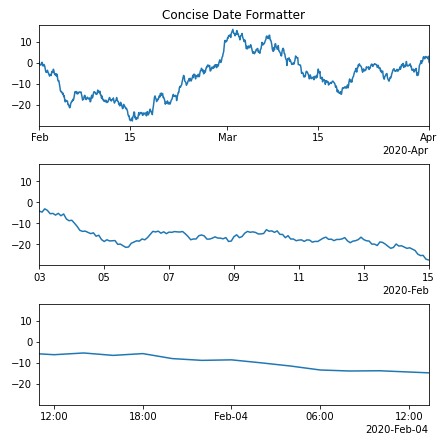Open in App
Not now

# Matplotlib.dates.ConciseDateFormatter class in Python

• Last Updated : 21 Apr, 2020

Matplotlib is an amazing visualization library in Python for 2D plots of arrays. Matplotlib is a multi-platform data visualization library built on NumPy arrays and designed to work with the broader SciPy stack.

## matplotlib.dates.ConciseDateFormatter

The `matplotlib.dates.ConciseDateFormatter` class is used to figure out the best format to use for the date and also makes it as compact as possible but complete. This is more often used with `AutoDateLocator`.

Syntax: class matplotlib.dates.ConciseDateFormatter(locator, tz=None, formats=None, offset_formats=None, zero_formats=None, show_offset=True)

Parameters:

• locator: This parameter represents the locator that this axis uses.
• tz: It is an optional parameter that accepts a string that is passed to dates.date2num.
• formats: It is an optional list of 6 strings. It is used to format strings for 6 levels of tick labelling as years, months, days, hours, minutes and seconds. These strings has format codes same as that of strftime. Its defaults are as [ ‘%Y’, ‘%b’, ‘%d’, ‘%H:%M’, ‘%H:%M’, ‘%S.%f’]
• zero_formats: It is an optional list of 6 strings. It is used for formatting strings for tick labels that are “zero” for any given tick level. For example, if most ticks are months, ticks around 1 February 2020 will get labeled “Jan” 2020 “March”.Its defaults are as [”, ‘%Y’, ‘%b’, ‘%b-%d’, ‘%H:%M’, ‘%H:%M’].
• offset_formats: It is an optional list of 6 strings.It is used for formatting strings for 6 levels that is applied to the “offset” string on the right side of x-axis or top of y-axis. This should completely specify date when combined with the tick labels. The defaults are as [”, ‘%Y’, ‘%Y-%b’, ‘%Y-%b-%d’, ‘%Y-%b-%d’, ‘%Y-%b-%d %H:%M’].
• show_offset: It accepts a boolean value and decides whether to show offset or not. By default it is set to True.
• Example 1:

 `import` `numpy as np``import` `matplotlib.dates as mdates``import` `matplotlib.pyplot as plt`` ` `  ` `# dummy date``dummy_date ``=` `np.arange(``"2020-04-10"``, ``                       ``"2020-05-14"``,``                       ``dtype ``=``"datetime64"``)`` ` `random_x ``=` `np.random.rand(``len``(dummy_date))``  ` `figure, axes ``=` `plt.subplots()`` ` `axes.plot(dummy_date, random_x)``axes.xaxis.``set``(``    ``major_locator ``=` `mdates.AutoDateLocator(minticks ``=` `1``,``                                           ``maxticks ``=` `5``),``)`` ` `locator ``=` `mdates.AutoDateLocator(minticks ``=` `15``,``                                 ``maxticks ``=` `20``)``formatter ``=` `mdates.ConciseDateFormatter(locator)`` ` `axes.xaxis.set_major_locator(locator)``axes.xaxis.set_major_formatter(formatter)``  ` `plt.show()`

Output:Example 2:

 `import` `datetime``import` `matplotlib.pyplot as plt``import` `matplotlib.dates as mdates``import` `numpy as np`` ` ` ` `dummy_date ``=` `datetime.datetime(``2020``, ``2``, ``1``)`` ` `# random date generator``dates ``=` `np.array([dummy_date ``+` `datetime.timedelta(hours ``=``(``2` `*` `i))``                  ``for` `i ``in` `range``(``732``)])``date_length ``=` `len``(dates)`` ` `np.random.seed(``194567801``)``y_axis ``=` `np.cumsum(np.random.randn(date_length))`` ` `lims ``=` `[(np.datetime64(``'2020-02'``), ``         ``np.datetime64(``'2020-04'``)),``        ``(np.datetime64(``'2020-02-03'``), ``         ``np.datetime64(``'2020-02-15'``)),``        ``(np.datetime64(``'2020-02-03 11:00'``), ``         ``np.datetime64(``'2020-02-04 13:20'``))]`` ` `figure, axes ``=` `plt.subplots(``3``, ``1``, ``                            ``constrained_layout ``=` `True``, ``                            ``figsize ``=``(``6``, ``6``))`` ` `for` `nn, ax ``in` `enumerate``(axes):``     ` `    ``locator ``=` `mdates.AutoDateLocator(minticks ``=` `3``, maxticks ``=` `7``)``    ``formatter ``=` `mdates.ConciseDateFormatter(locator)``     ` `    ``ax.xaxis.set_major_locator(locator)``    ``ax.xaxis.set_major_formatter(formatter)`` ` `    ``ax.plot(dates, y_axis)``    ``ax.set_xlim(lims[nn])``     ` `axes[``0``].set_title(``'Concise Date Formatter'``)`` ` `plt.show()`

Output:My Personal Notes arrow_drop_up